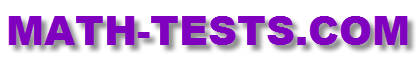#Take this 3rd Grade Division Test to check your division skills and your ability to solve word problems.

The quiz has ten problems: seven multiple choice, and three drag and drop/matching problems.
Third grade students will investigate how division and multiplication are related, divide using related multiplication facts, explore division as equal sharing, and solve word problems about division.

This test is based on the following Common Core Standards:

CCSS.Math.Content.3.OA.A.2
Interpret whole-number quotients of whole numbers.

CCSS.Math.Content.3.OA.A.3
Use multiplication and division within 100 to solve word problems.

CCSS.Math.Content.3.OA.B.6
Understand division as an unknown-factor problem.

CCSS.Math.Content.3.OA.C.7
Fluently multiply and divide within 100.